Core Java - Interview Questions and Answers for 'Code optimization' | Search Java Interview Question - javasearch.buggybread.com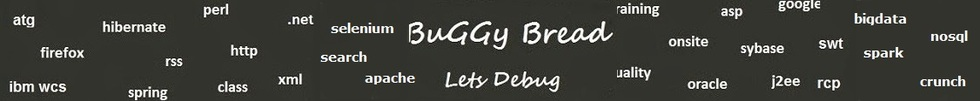# Search Interview QuestionsMore than 3000 questions in repository.There are more than 900 unanswered questions.Have a video suggestion.
Click Correct / Improve and please let us know.
Label / Company      Label / Company / TextSubmit Question

Core Java - Interview Questions and Answers for 'Code optimization' - 3 question(s) found - Order By Newest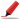This question can be asked in different variants like performace improvement of an application, improving performance for DB communication etc.Q1. What are the ways in which execution time of the code can be improved ?Solution2016-05-29 09:42:39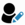Ans. This depends on entirely upon the code type. For example

1.If its just logic, we can try it to short circuit or put the if / switch case with maximum probability in the beginning.

2. Can use faster data structures, for eg - random retrieval instead of sequence / iterator

3. Working with primitive types or even bytes instead of Objects, even though it may result in marginal improvement.

4. If its service call, then service call with bulk load can help

5. If DB Operation, then with use of Indices , Views or using ORM , cache etc.Help us improve. Please let us know the company, where you were asked this question :LikeDiscussCorrect / Improveperformance improvement  code optimization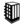Asked in 1 CompaniesRelated Questions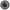What things you would care about to improve the performance of Application if its identified that its DB communication that needs to be improved ?Q2. What is wrong with the following if statement ?

if(x==y && x.equals(y) {
}
Core Java2016-07-28 13:45:21Ans. x==y means that both references have same type and are pointing to same memory location and hence would always mean that they have same value.

x.equals(y) is not required in this case.Help us improve. Please let us know the company, where you were asked this question :LikeDiscussCorrect / Improveif statement  control statements  == and equals  ==  .equals   code optimization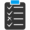Try 2 Question(s) TestRelated QuestionsDifference between == and .equals() ?What is the difference between = and == in Java ?How is == operator different for objects and primitive types ?What will be the output of following code

Integer x = 1;
Integer y = 2;
System.out.println(x == y);

What if you change 1 to "1" and Integer to String?Q3. What is wrong with the following if statement ?

if(x==y || x.equals(y) {
}
Core Java2016-07-28 13:48:23Ans. if x==y turns out to be true x.equals(y) will be true too. If x.equals(y) could be true even if x==y is true or not.

So the only possible outcomes are

1 || 1 = 1
0 || 1 = 1
0 || 0 = 0

i.e the outcome of x.equals(y)

check for x==y is not required in this if statement.Help us improve. Please let us know the company, where you were asked this question :LikeDiscussCorrect / Improveif statement  control statements  == and equals  ==  .equals   code optimizationTry 1 Question(s) TestRelated QuestionsDifference between == and .equals() ?What is the difference between = and == in Java ?How is == operator different for objects and primitive types ?What will be the output of following code

Integer x = 1;
Integer y = 2;
System.out.println(x == y);

What if you change 1 to "1" and Integer to String?

## Help us and Others Improve. Please let us know the questions asked in any of your previous interview.

Any input from you will be highly appreciated and It will unlock the application for 10 more requests.